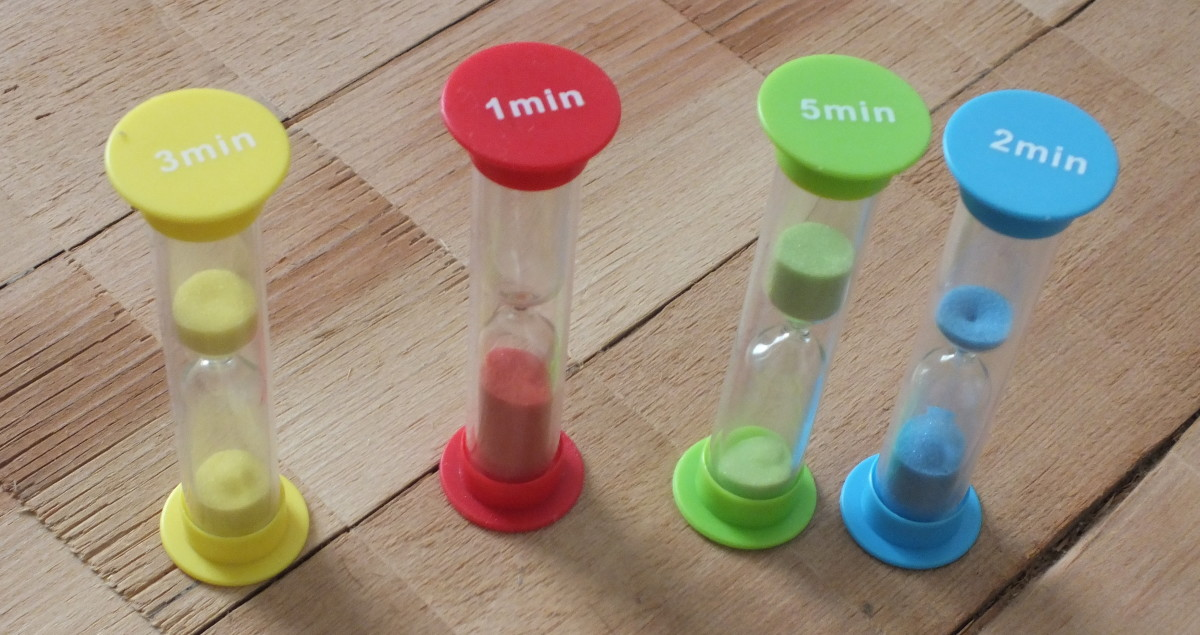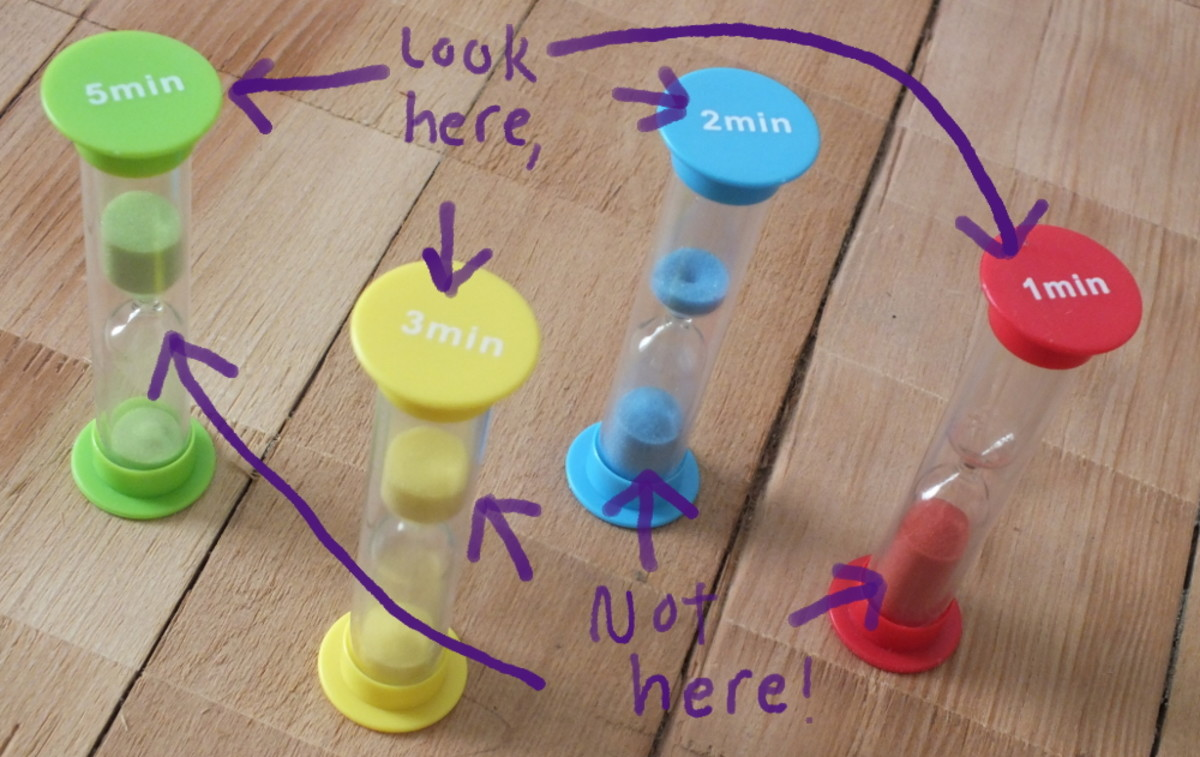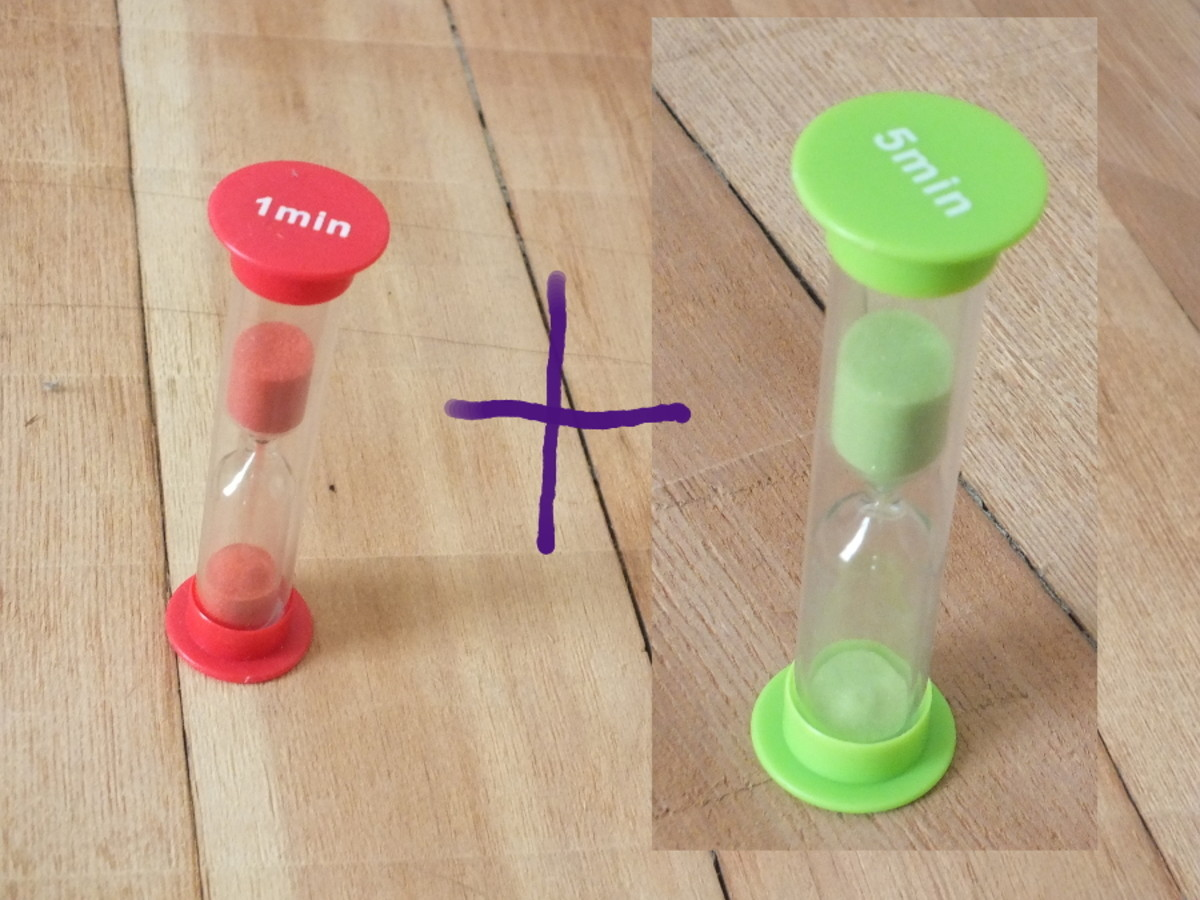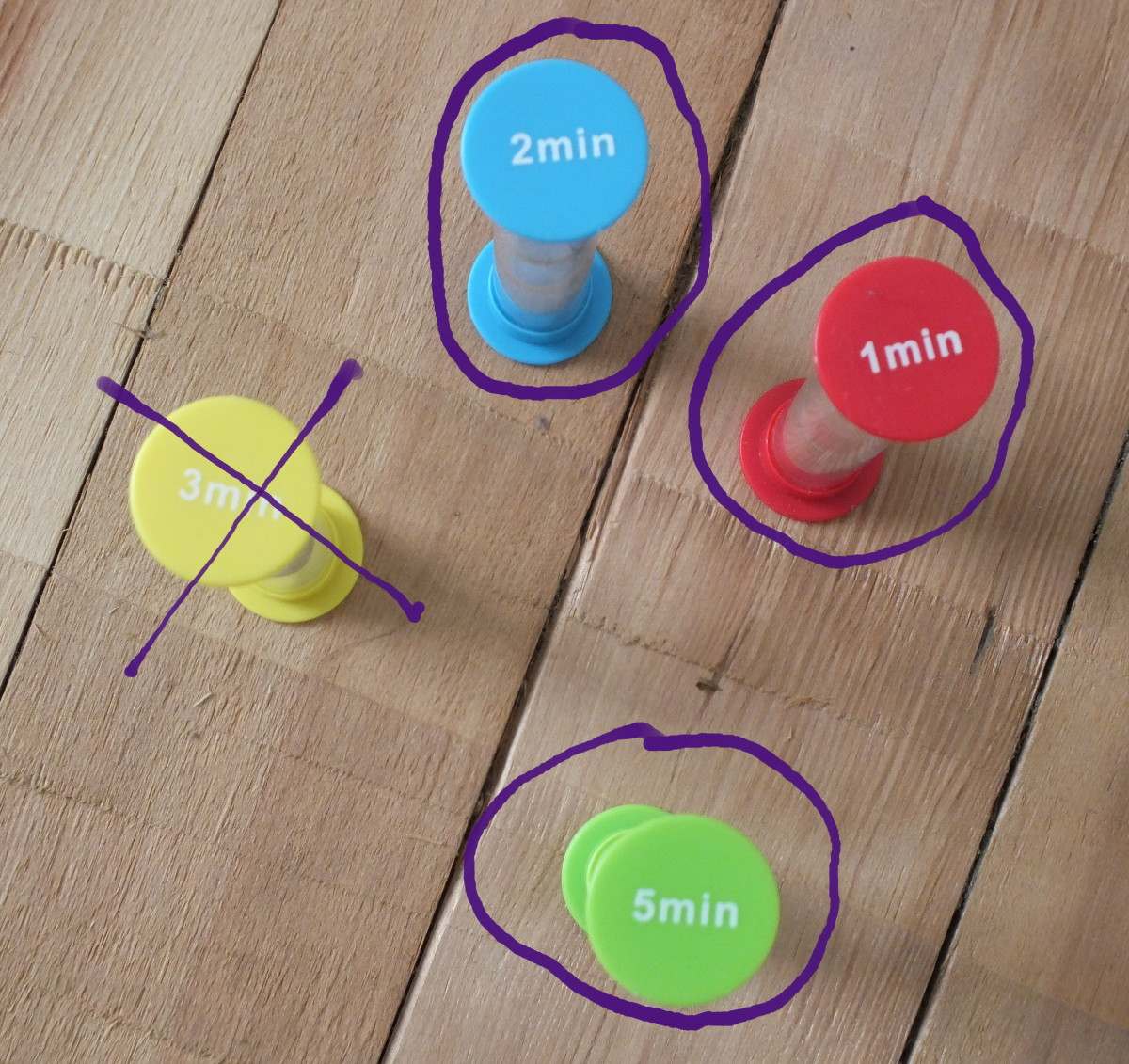# Math & Logic Games With Sand Timers for Kids

Chris Telden's B.A. degree in sociology focused on effective quantitative and qualitative methods of cultural analysis.

When my first-grader was given a 2-minute timer at school to help him learn how to brush his teeth, he asked for more. I got him a set of four hourglass sand timers that I sneakily planned to use as a learning tool for mathematics and logic. Here are some of the simple exercises we did. The exercises start easy, then get progressively harder. Educators and parents can guide young children through these problems to help get them thinking about mathematical concepts and problems of logic.

## Supplies You Need

For this series of exercises, you need a matching set of four sand timers containing 1-minute, 2-minute, 3-minute, and 5-minute timers. I used the inexpensive Teacher Created Resources set as they are of decent quality and are made of plastic, not glass.

The set you use can include different colors or be just one color. However, the sizes and shapes should be the same, or these logic problems could get confusing. (Later on, for more of a challenge, you can try using timers that don't match.)Green, Red, Blue, and Yellow Sand Timers for Kids Measuring 1, 2, 3, and 5 MinutesChris Telden

## Logic Experiment Set-Up

These hourglass sand timers are measuring devices, like rulers. They don't measure inches or centimeters, though. Instead, they measure time in units called "minutes." As you may know, each minute is 60 seconds long.

Look at the sand timers and identify how much time each one takes to run. Go through each timer and test it against a clock. Check to see if they run at exactly the correct times: 1 minute, 2 minutes, 3 minutes, 5 minutes. If so, great! You're set to go. If not - you know what? That's all right for our purposes. Let's just pretend they are accurate and get started!To figure out which timer is which, look at their labels, not how much sand is in them.Chris Telden

## Logic Question Set 1 - Easy

These basic questions will get you acquainted with the timers and what they do.

1. If you wanted to brush your teeth for two minutes, and you had to use just one timer, which timer would you use?
2. If you had only five minutes to play a game, and you had to use just one timer, which timer would tell you when to stop?
3. If you wanted to run around for three minutes, and you had to use just one timer, which timer would you pick? If you were allowed to use more than one timer to time those three minutes, could you? Which ones would you use, and why?
4. If a friend said to you, "Meet me there in one minute," which timer would you use? Is there any other timer you could use besides that one? Why or why not?

For answers, see the section at the end.

## Logic Question Set 2 - Harder

Let's say your parent said to you, "I want you all dressed and ready to go in six minutes!" Think about the different ways you could use these timers to help you be ready in exactly six minutes.

1. Could you measure exactly six minutes with only one timer? How would you do it? What about with only two timers? What about three? Or four?

Write down as many combinations as you can that would get you six minutes. (See the end of this page for ALL the answers - and there are a LOT.)

## Hint, Hint...Use this hint to figure out how to measure 6 minutes.Chris Telden

## Logic Questions Set 3 - Even Harder

Let's say you had just ten minutes to work on math homework, but you only had one timer to help you time yourself.

1. Which timer(s) could you use?
2. Which timer(s) could you NOT use?

Scroll to Continue

## Logic Questions Set 4 - Hardest

This problem set is about logic as much as it is about math - even though math, itself, is all about logic. (Logic is when you have something that you know is true and because it is true, something else has to be true and something else has to be false.)

Recall that hourglass sand timers measure time in units of minutes. Look at your timers and answer these questions.

1. If you add up all of the minutes the timers measure together, what number do you get?
2. How would you get the timers to tell you when that many minutes (the number you came up with in the previous question) have passed?
3. Let's say you just have the 1-minute timer but not the others. How would you get it to tell you that two minutes have passed?
4. Now let's say you just have the 2-minute timer but not the others. How would you get it to tell you that four minutes have passed?
5. Now let's pretend you only have two timers, and they are both 2-minute timers. If you add up the minutes that they measure, what number do you get?
6. Take those two 2-minute timers from the last question. What would happen if you ran them one after the other?
7. Now take those two 2-minute timers one more time. What would happen if you ran them together at the same time? How many minutes would they measure?

Find all the answers to these questions below.

## Bonus Question

You might wonder how hourglass timers work. We can all see the sand flowing through the hourglass, but how do the timers know to run for one, two, three, or five minutes?

Do you think the amount of sand makes a difference? We can test it by examining the timers. Does the 5-minute timer have five times as much sand as the 1-minute timer?

What else can affect how quickly or slowly the sand flows through the timer?

Study the timers and figure it out! This is truly a bonus question - no answer provided!

The following section has the answer key to the logic sets. Use this if you don't know, or aren't sure of, the answers to any questions. Even if you do know, some of the answers can be fun to read.

## Answers to Logic Question Set 1

1. The 2-minute timer.
2. The 5-minute timer.
3. The 3-minute timer. You could use other timers if their run time together adds up to three minutes. So you could use the 1-minute timer with the 2-minute timer, because their run time together adds up to exactly three minutes.
4. The 1-minute timer. You could not use any other timer, because all the other timers take longer than one minute.

## Answers to Logic Question Set 2

It is possible to measure six minutes exactly with only one timer. The way you measure six minutes with one timer is to run the timer again and again until you've reached six minutes.

You can also use two or three timers and run them in different combinations, one after the other.

You cannot use all four timers in a single sequence to measure six minutes, because if you are using the 5-minute timer, the other timers will make your total number of minutes too great.

In the following chart you'll find all the possible combinations of timers and sequences that you could run to measure six minutes with these timers. There are 25 different sequences in all, with 8 different timer combinations.

All the possible combinations of sand timer runs to yield 6 minutes.

Timer Sequences to UseExplanation

5+1, 1+5

Run the 5-minute timer first, then immediately run the 1-minute timer. Or do it the other way around and run the 1-minute timer first, then the 5-minute timer.

3+3

Run the 3-minute timer two times in a row.

2+2+2

Run the 2-minute timer three times in a row.

1+2+3, 1+3+2, 3+2+1, 3+1+2, 2+1+3, 2+3+1

Run the 1, 2, and 3-minute timers one right after the other. You can run them in any order as long as for each series, you run each timer only once.

1+1+1+1+1+1

Run the 1-minute timer six times in a row.

1+1+1+3, 1+1+3+1, 1+3+1+1, 3+1+1+1

Run the 1-minute timer 3 times and the 3-minute timer one time, in any order.

1+1+1+1+2, 1+2+1+1+1, 1+1+2+1+1, 1+1+1+2+1, 2+1+1+1+1

Run the 1-minute timer 4 times and the 2-minute timer once, in any order.

1+1+2+2, 2+2+1+1, 1+2+1+2, 1+2+2+1, 2+1+1+2

Run the 1-minute timer twice and the 2-minute timer twice, in any order.

## Answers to Logic Question Set 3

1. For this problem, you need to use multiplication. That is because you only have one timer. If you had more timers, you could just use addition. You would add a few minutes here and a few minutes there until you have exactly the sum of ten. But with just one timer, the only way to make the minutes of each timer "grow" into ten minutes is to multiply its minutes by some number of runs. So you could use the 1, 2, or 5-minute timers because 1, 2, and 5 are all factors of ten. You could run the 1-minute one ten times (that's 1 x 10), or the 2-minute one five times (that's 2 x 5), or the 5-minute one two times (5 x 2).
2. You could not use the 3-minute timer, because three is not a factor of 10. If you ran the timer once, you'd just get three minutes. Twice would get you six minutes. Three times would get you nine minutes. Four times would get you 12 minutes. So ten minutes would just be "skipped over." If your only timer were a 3-minute timer, you'd either end up with too few minutes or too many.The 3-minute timer is the only one that doesn't factor in to 10.Chris Telden

## Answers to Logic Questions Set 4

1. The sum of the minutes the timers measure is 11. You get this from adding 1+2+3+5.
2. To get the timers to tell you that 11 minutes have passed, you run them sequentially. Sequentially is another way of saying "one after another." So you would run each of your timers - the 1-minute timer, the 2-minute timer, the 3-minute timer, and the 5-minute timer - once each, in any order, right after the other. At the end, 11 minutes will have passed.
3. Run the 1-minute timer twice in a row.
4. Run the 2-minute timer twice in a row.
5. Four. Each timer measures 2 minutes. 2+2=4.
6. You would run through a 4-minute interval.
7. This is a trick question. You would NOT run through a 4-minute interval. You would only run through two minutes. That is because if you ran both timers at the same time, you are running them through the same two minutes. You cannot measure the same minute twice. To help see why this is, imagine that each 2-minute timer is in a different room with a different person. You tell each person "Okay, start your timers now! Call out when your timer is done!" So at the end of two minutes, each person calls out, "My timer is done!" Even though four minutes were measured - two in one room, and two in the other - both hourglass sand timers were measuring the same two minutes. Another way to think of it is this: An hourglass sand timer is a measuring device. So is a ruler. Let's look at that ruler. If you measure an inchworm with a ruler, you might find it is an inch long. If you measure an inchworm with two rulers, it's still an inch long. But you measured an inch twice. That doesn't make it two inches long. So when you use two 2-minute timers at the same time, you're using them to measure the same two minutes. But it's still two minutes and not four.

## Disclosure

Chris Telden (author) from Pacific Northwest, U.S.A. on March 04, 2015:

Pstraubie48, that must be a blast. Ever notice how as far as kids are concerned, the inexpensive gadgets and geegaws go as far, if not farther, than the pricey learning sets...?

Chris Telden (author) from Pacific Northwest, U.S.A. on March 04, 2015:

RTalloni, you're welcome! I had a good time with the kid, and I think we've only tapped the potential of these timers. I think the simple machines are awesome for teaching basic concepts about our world. ;)

Patricia Scott from North Central Florida on March 04, 2015:

Clever use for these timers. My grandson has a collection of them that I got for him and he loves it as we used them for a variety of fun (learning) games.

Pinned voted up+++ shared g+

Angels are on the way to you this afternoon. ps

RTalloni on March 03, 2015:

Thanks for sharing these kid-level ideas for expanding their thinking skills by working with timers. Neat hub all round!